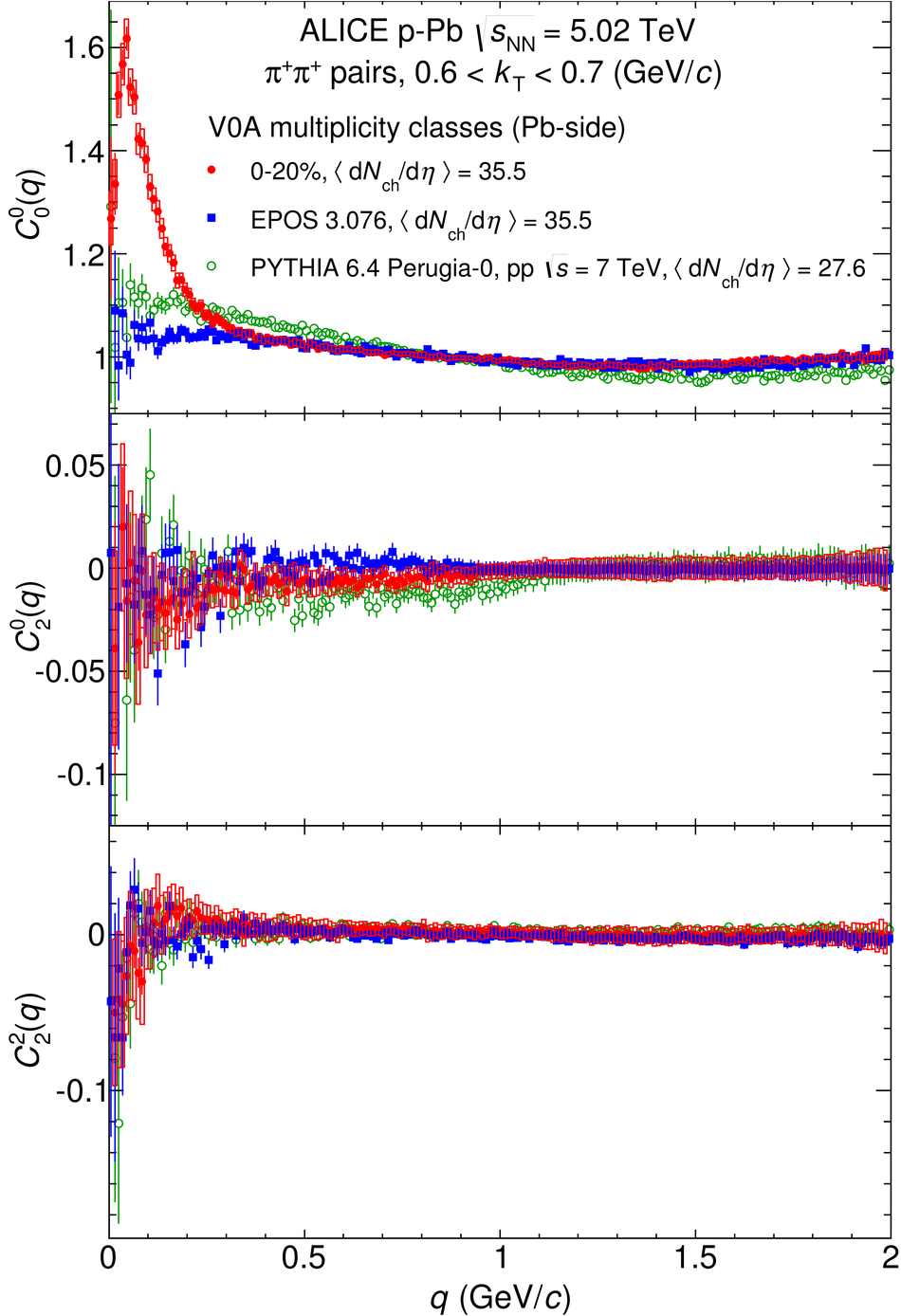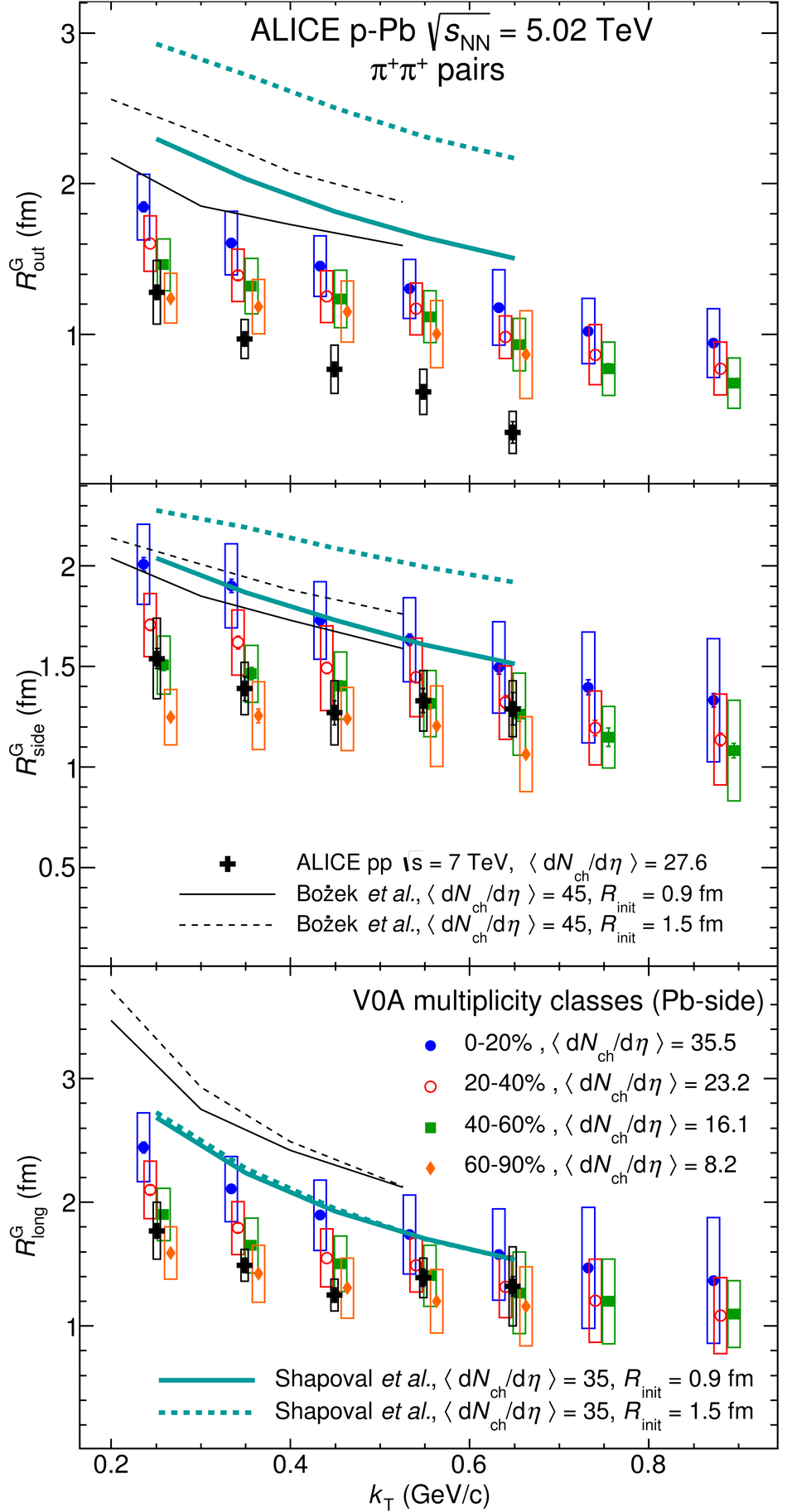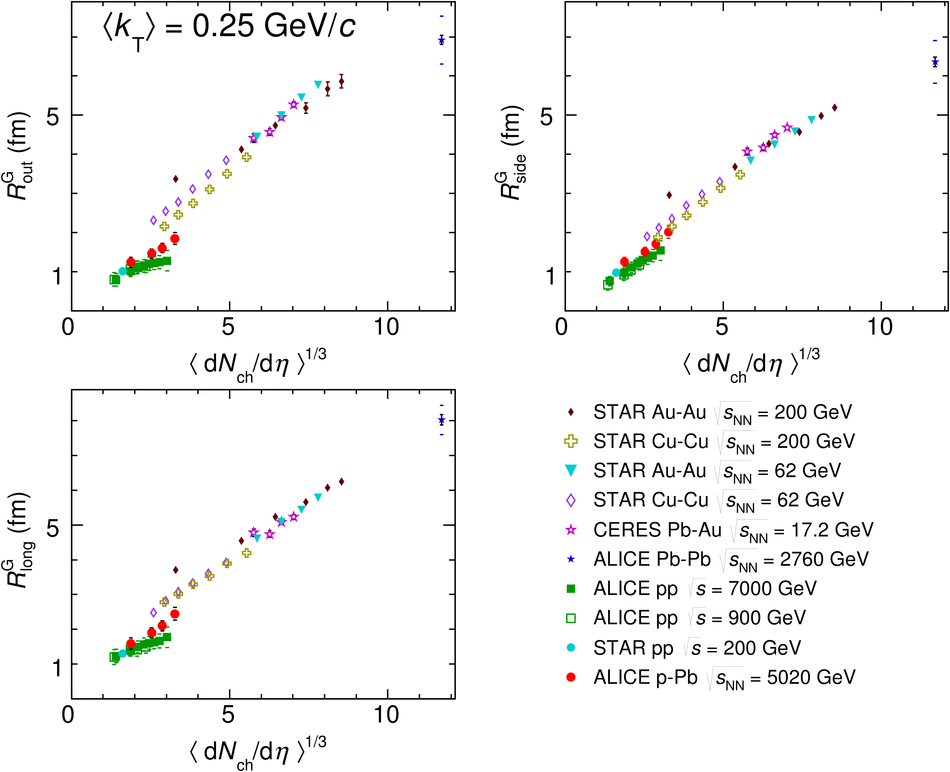# Two-pion femtoscopy in p-Pb collisions at $\sqrt{s_{\rm NN}}=5.02$ TeV

We report the results of the femtoscopic analysis of pairs of identical pions measured in p-Pb collisions at $\sqrt{s_{\mathrm{NN}}}=5.02$ TeV. Femtoscopic radii are determined as a function of event multiplicity and pair momentum in three spatial dimensions. As in the pp collision system, the analysis is complicated by the presence of sizable background correlation structures in addition to the femtoscopic signal. The radii increase with event multiplicity and decrease with pair transverse momentum. When taken at comparable multiplicity, the radii measured in p-Pb collisions, at high multiplicity and low pair transverse momentum, are 10-20% higher than those observed in pp collisions but below those observed in A-A collisions. The results are compared to hydrodynamic predictions at large event multiplicity as well as discussed in the context of calculations based on gluon saturation.

Figures

## Figure 1

 Projections of the three-dimensional $\pi^+\pi^+$ correlation functions for three selected multiplicity and $k_{\rm T}$ ranges along the $out$ (top), $side$ (middle), and $long$ (bottom) direction. The other components are integrated over the four bins closest to zero in their respective $q$ directions## Figure 2

 First three non-vanishing components of the SH representation of the $\pi^+\pi^+$ correlation functions for three multiplicity and $k_{\rm T}$ ranges, $l=0$, $m=0$ (top), $l=2$, $m=0$ (middle), and $l=2$, $m=2$ (bottom).## Figure 3

 Dependence of the SH components of the correlation function on event multiplicity in a broad relative momentum range.## Figure 4

 First three non-vanishing components of the SH representation of the $\pi^+\pi^+$ correlation functions for a selected multiplicity and $k_{\rm T}$ range, compared to a calculation from EPOS 3.076 (generator level only) and PYTHIA 6.4 Perugia-0 tune for pp at $\sqrt{s}=7$ TeV (full simulation with detector response)## Figure 5

 First three non-vanishing components of the SH representation of the $\pi^+\pi^+$ correlation functions for three multiplicity and $k_{\rm T}$ combinations, $l=0$, $m=0$ (top), $l=2$, $m=0$ (middle), and $l=2$, $m=2$ (bottom). The lines show the corresponding components of the Gaussian (GGG) fit.## Figure 6

 First three non-vanishing components of the SH representation of the $\pi^+\pi^+$ correlation functions for three multiplicity and $k_{\rm T}$ combinations, $l=0$, $m=0$ (top), $l=2$, $m=0$ (middle), and $l=2$, $m=2$ (bottom). The lines show the corresponding components of the EGE fit.## Figure 7

 Femtoscopic radii (GGG fit) as a function of the pair transverse momentum $k_{\rm T}$ for four multiplicity classes. For comparison, radii from high-multiplicity pp collisions at $\sqrt{s}=7$ TeV and 4 predictions for p-Pb are shown as crosses and lines, respectively. Top, middle, and bottom panel show $R_{\mathrm{out}}$, $R_{\mathrm{side}}$, and $R_{\mathrm{long}}$ radii, respectively. The points for multiplicity classes 20-40% and 40-60% have been slightly shifted in $k_{\rm T}$ for visibility.## Figure 8

 Femtoscopic radii (EGE fit) as a function of the pair transverse momentum $k_{\rm T}$ for four multiplicity classes. For comparison, radii from high-multiplicity pp collisions at $\sqrt{s}=7$ TeV are shown. Top, middle, and bottom panel show $R^{E}_{out}$, $R^{G}_{side}$, $R^{E}_{long}$ radii, respectively. The data points for the multiplicity classes 20-40% and 40-60% have been slightly shifted in $k_{\rm T}$ for visibility.## Figure 9

 Comparison of femtoscopic radii (Gaussian), as a function of the measured charged-particle multiplicity density, measured for various collision systems and energies by CERES, STAR, PHENIX, and ALICE.